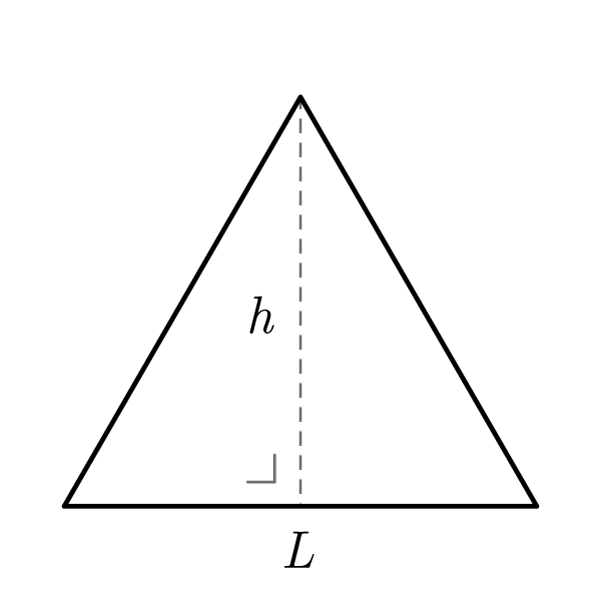# Equilateral Triangle

 Side $$S$$ Height $$h$$
$$2p = S \times 3$$
Perimeter
$$A = \frac{S \times h}{2}$$
Area
$$S = \frac{A \times 2}{h}$$
$$h = \frac{A \times 2}{S}$$
$$S = \frac{2p}{3}$$
Side
Knowing side or height only
$$2p = 2 h \sqrt{3}$$
Perimeter (with height)
$$h = \frac{2p}{2\sqrt{3}}$$
Height (from perimeter)
$$A = \frac{\sqrt{3}}{4} {S}^2$$
Area (with side)
$$A = \frac{{h}^2}{\sqrt{3}}$$
Area (with height)
$$S = \frac{2h}{\sqrt{3}}$$
Side (with height)
$$h = \frac{S\sqrt{3}}{2}$$
Height (with side)
Inscribed circumference
$$r = \frac{1}{2 \sqrt{3}} S$$
Inscribed radius (with side)
$$r = \frac{1}{3} h$$
Inscribed radius (with height)
$$S = 2r\sqrt{3}$$
Side
$$h = 3r$$
Height
$$2p = 6r\sqrt{3}$$
Perimeter
$$A = 3{r}^2 \sqrt{3}$$
Area
Circumscribed circumference
$$R = \frac{\sqrt{3}}{3} S$$
Circumscribed radius (with side)
$$R = \frac{2}{3} h$$
Circumscribed radius (with side)
$$S = R\sqrt{3}$$
Side
$$h = \frac{3}{2}R$$
Height
$$2p = 3\sqrt{3}R$$
Perimeter
$$A = \frac{3\sqrt{3}}{4}{R}^2$$
Area

## Definition

An equilateral triangle is a triangle with all sides congruent.

## Properties

1. All sides are congruent
2. All angles are congruent
3. All the  Generic Triangle formulas are valid
4. The height relative to the base divides the shape in two congruent right triangles. For these are valid the  Right Triangle formulas## Equilateral Triangle Formulas

Data Formula
Perimeter 2p = S × 3
Area A = (S × h) / 2
Side S = 2p / 3
Side (with height) S = (2 × h) / √3
Height (with side) h = (S√3) / 2
Height (from perimeter) h = 2p / (2√3)
Perimeter (with height) A = 2 × h × √3
Area (with side) A = (√3 / 4) × S2
Area (with height) A = h2 / √3
Side S = (A × 2) / h
Height h = (A × 2) / S

## Equilateral Triangle - Inscribed circumference

Data Formula
Inscribed radius (with side) r = [1 / (2√3)] × S
Inscribed radius (with height) r = 1/3 × h
Side S = 2r√3
Height h = 3r
Perimeter 2p = 6r√3
Area A = 3r2√3

## Equilateral Triangle - Circumscribed circumference

Data Formula
Circumscribed radius (with side) r = (√3 / 3) × S
Circumscribed radius (with height) r = 2/3 × h
Side S = R√3
Height h = 3/2 × R
Perimeter 2p = 3√3R
Area A = [(3√3) / 4] × R2